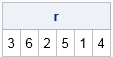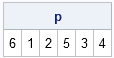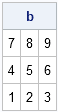I recently read a paper that described a SAS macro to carry out a permutation test. The permutations were generated by PROC IML. (In fact, an internet search for the terms "SAS/IML" and "permutation test" gives dozens of papers in recent years.) The PROC IML code was not as efficient as it could have been, so this post discusses how to efficiently generate random permutations and how to use them to permute rows of a matrix.

To review, a permutation is a reordering of the elements of a vector. For example, one permutation of the set {1 2 3 4} is the vector {2 1 4 3}.

### Use the RANPERM function in SAS/IML 9.3

If you are using SAS 9.3, you can use the RANPERM function in the SAS/IML language. For example, suppose you want a random permutation of the set {1 2 3 4 5 6}. You can use the RANPERM function as follows:

```proc iml; call randseed(123); r = ranperm(6); print r; quit;```### Random permutations without using RANPERM

If you don't have access to SAS 9.3, you can still generate a random permutation. One way is to generate N random numbers from the uniform distribution and then use the RANK function to enumerate the order of the random numbers.

For example, suppose you want a random permutation of the set {1 2 3 4}. You can generate a vector of four random numbers such as u = {0.34 0.12 0.78 0.56} and compute rank(u), which is the vector {2 1 4 3}.

The following statements define a SAS/IML module that returns a random permutation of N elements:

```proc iml; /** generate one random permutation with N > 0 elements **/ start RandPerm(N); u = 1:N; /** allocate u **/ call randgen(u, "Uniform"); /** fill with random numbers **/ return(rank(u)); /** return rank (order) of elements **/ finish;   /** call the permutation module **/ call randseed(123); /** optional: make numbers reproducible **/ p = RandPerm(6); print p;```### Random permutations of rows

You can use permutations in many ways. For example, you might want to permute the rows of a matrix as part of a bootstrap computation or some other resampling technique. The following statements define a module that permutes the rows of a matrix:

```/** Module to permute the rows of a matrix **/ start PermuteRows(M); n = nrow(M); /** how many rows? **/ r = RandPerm(n); /** or use RANPERM in SAS 9.3 to get a random permutation **/ return ( M[r,] ); /** return matrix with permuted rows **/ finish;   /** test the module **/ a = {1 2 3, 4 5 6, 7 8 9}; b = PermuteRows(a); print b;```Share1.